## Tuesday, March 10, 2015

### ## Derivatives and regression again

I've been writing about how a "sliding" trend may function as a estimate of derivative (and what might be better) here, here, here and here. There has been discussion, particularly commenter Greg. This post just catches up on some things that arose.

#### Does it differentiate?

Greg is not so sure. I'll give some examples. First here is a graph of some of the windows. I've added a step function, to show that in fact virtually any odd function will differentiate. The one called Welch^2 is the linear window multiplied by the square of a Welch taper. There is information about the Welch taper, and why it arises naturally here, here.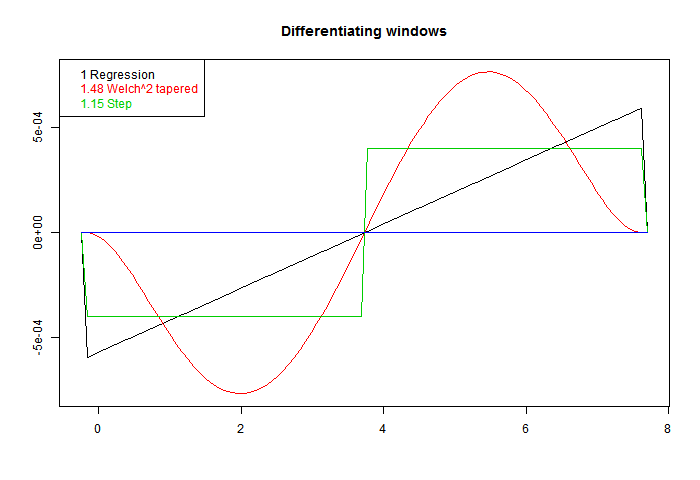These are scaled to give correct derivative for a line. On the legend, I have written beside each the RMS value. Sqrt of the integral of square. That's the factor that you would expect to multiply white noise, as a variance of weighted sum. Ordinary regression has the least value. That is an aspect of its optimal quality. Better smoothing for HF comes at a cost.

So now to apply these to a sinusoid. In the following plot the yellow is the sine to be differentiated, the continuous curve "Deriv" is the exact derivative, and I have applied the three windows with three different footprints (line types). At each end, a region equal to half a footprint is lost - I have marked those parts with a horizontal line, which tells you how long each footprint was.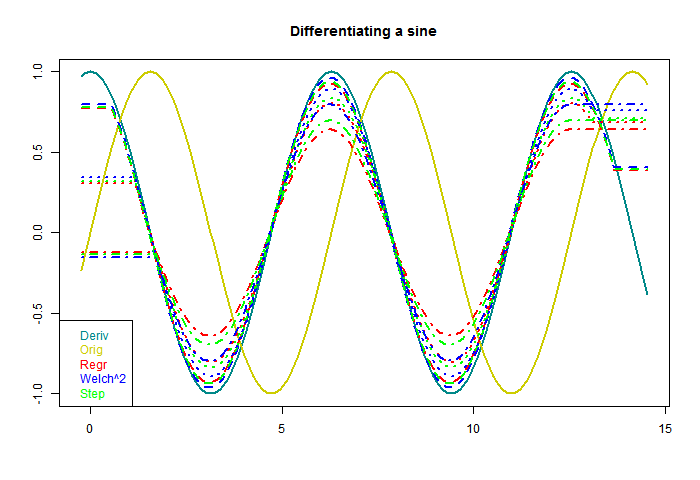The first thing to note is that each gives a sinusoid, with the correct 90° phase shift. The is a consequence of being an odd function. Second is that as the footprint broadens, the amplitude shrinks. The inaccuracy is because a wider footprint is more affected by higher derivatives of the sine. In effect, it smoothes the result, to no good purpose here. But when there is noise, the smoothing is needed. That is the basic trade-off that we will encounter.

You'll notice, too, that both the Welch and step do better than regression. This is basically because they are weighted to favor central values, rather than any more subtle merit.

Now for something different, to differentiate white noise. There should be no real trend. I've dropped the step filter. Again the horizontal end sections indicate the half-footprint.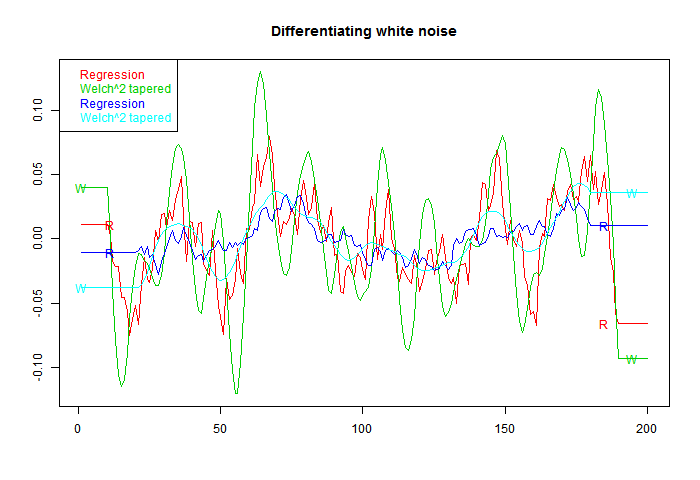Now what shows is a marked oscillation, with period about equal to the footprint. The Welch filter is good at damping frequencies beyond this range; however, the actual amplitude of the response is much higher. That is associated with the higher RMS value noted on the first figure, and is commensurate with it.

#### Integrating with noise

OK, so what happens if we estimate the derivative of a sinusoid with noise. The next fig has sine with gaussian noise of equal amplitude added. Can we recover the derivative?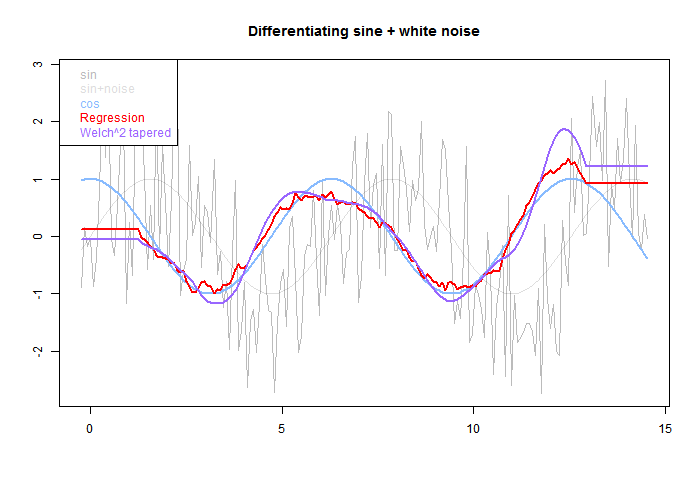The pale grey is the original, sine and total. The blue is the derivative of sine. The red OLS regression tracks better than the smoother purple, but has more residual HF. Again the half-footprint is shown by the level sections at each end.

#### Improving the derivative formula

Pekka, on another thread, recommended taking pairs of points, differencing, and forming some kind of optimal derivative as a weighted sum. Odd function windows automatically give such a weighted sum. The idea of improving the derivative (higher order) is attractive, because it allows the footprint to be expanded, with better noise damping, without loss of derivative accuracy.

A way to do this is to take a family of windows, and make them orthogonal to higher powers by Gram-Schmidt orthogonalisation. I did that using OLS, OLS with Welch taper, and OLS with Welch^2 (W0,W1,W0). Here are the resulting windows. W1 is guaranteed to be accurate for a cubic, and W2 for fifth order: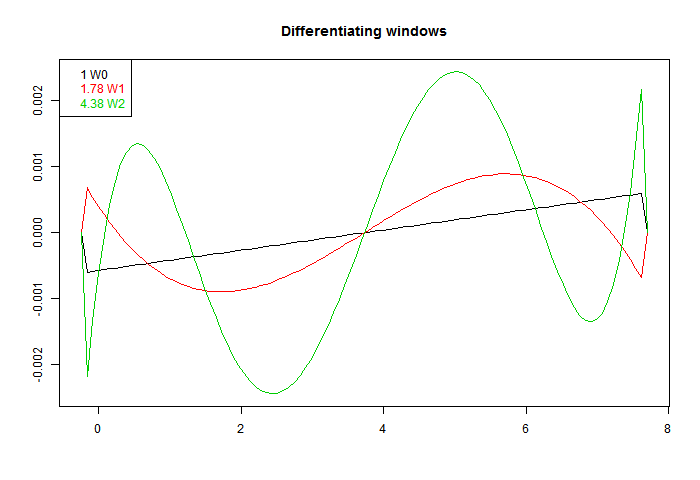Again, they are scaled to give the same derivative. We see a more extreme version of the RMS inflation of the first fig. The higher order accuracy is got at a cost of much larger coefficients. They do indeed differentiate better, as the next plot shows: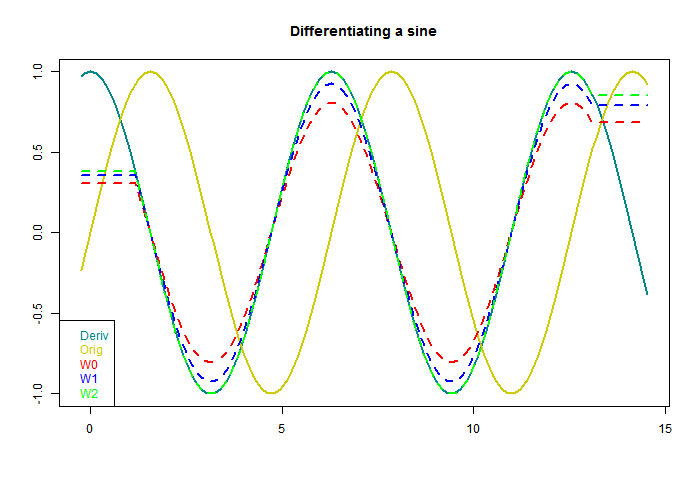W2 seems suspiciously perfect. But each result is just the correct sinusoid multiplied by a scalar, and I think it just approaches 1 in an oscillatory way, so W2 just happens to be a zero. Anyway, here higher order certainly works. What about with white noise?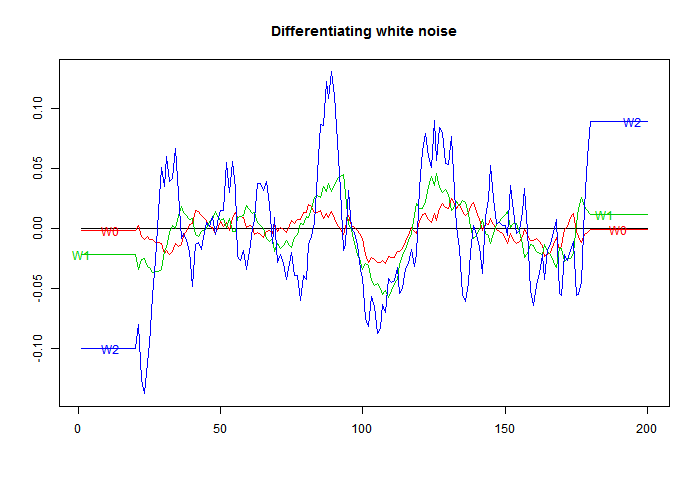Here is the downside. The higher orders amplify noise, in line with their RMS integrals.

Higher order may sometimes work. If you have an exact cubic, then you can expand the footprint as far as data permits without loss of derivative accuracy, and thus maybe overcome the RMS loss. But generally not.

1.Nick,

Your examples make it easier to explain, what I meant by an optimal choice.

One more example could be formed by adding to the linear and quadratic terms that allow for an unbiased estimate of the derivative a third order term of unknown strength. Now we assume that the coefficient of the third order term is normally distributed with a known standard deviation and expectation value zero. We assume also that noise is white and also normally distributed. We assume furthermore that all higher order odd terms are negligible.

Based on the above assumptions it's surely possible to determine a filter that leads to the smallest uncertainty for the derivative.

1.If the noise is white and uncorrelated, OLS gives you the unbiased filter design with the smallest unexplained variance (Gauss-Markshov theorem).

2.Should have said "linear unbiased filter design with the smallest unexplained variance".

You can sometimes do better with nonlinear processing.

3.Let's take a simple example of what I mean.

y is a function of x. We assume that the relationship is

y = Ax + Bx^3 + e

where A is the coefficient we wish to determine (derivative at x = 0). B is an unknown cubic term and e white noise.

To make the case simple we assume that the values of y have been determined at x = -2, -1, 0, 1, and 2. I denote these values by y[-2], y[-1], .., y.

We assume further that the uncertainty in B is represented by normal distribution with expectationn value of 0 and standard deviation of 1.0, and that also the white noise has the standard deviation of 1.0.

What are the optimal coefficients for estimating the coefficient A under these conditions?

The problem is relatively easy to solve (but I may have made an numerical error in my calculation). The result that I got is

A = 97/154 (y - y[-1]) - 5/77 (y - y[-2])

An alternative case is that where the two sources of uncertainty are equal at x = -2 and x = 2. In this case the stdev of B is 1/8 of that of the noise. In this case the formula is

A = 20/73 (y - y[-1]) + 33/292 (y - y[-2])

In the limit of very small uncertainty in B, we get the OLS formula

A = 1/10 (y - y[-1]) + 1/5 (y - y[-2])

and in the opposite limit, where white noise is insignificant

A = 2/3 (y - y[-1]) - 1/12 (y - y[-2])

My point is not that any one of these particular formulas were of obvious practical value, but just that specific assumptions on the sources of uncertainty lead to specific coefficients. When case the uncertainty due to the cubic term dominates at x = -2 and x = 2, these values are more significant for the estimation of the cubic coefficient, because I assumed that higher than cubic terms are not present. When such assumptions cannot be justified are cefficients should be positive, byt the relative weights do still depend on what we assume about the sources of uncertainty.

2."Now what shows is a marked oscillation, with period about equal to the footprint. The Welch filter is good at damping frequencies beyond this range; however, the actual amplitude of the response is much higher."

This is not an oscillation, it is the band of frequencies that you chose to let through. It is not amplifying, it is attenuating less. All the elements of the kernel should sum to unity. So everything gets attenuated, some things less than others. Nothing should be amplified.

As Carrick pointed out, the average is the best linear solution to remove normally distributed *random* noise. The problem is that it is not the best way to design a filter in the presence of non-random variability.

Thus again, the need to define the objective before choosing the solution.

If you have truly random noise, a mean is usually the best way to remove it. But this is were climatologists usually fail. They want to find CO2 forcing and dismiss the rest of climate variability as random "internal variability". They may say "stochastic" is they want to stop people arguing back.

Random becomes an excuse for anything that cannot be explained by CO2

Then linear trends and running means are applied across the board.

The trouble is, as we saw with the volcanoes and 15y sliding trends, it messes up badly. Volcanoes may be random in the sense that we do not have enough knowledge to predict them but three major stratospheric events with climatic impacts lasting about 4 or 5 years each in 1963, 1982,1991 does not look that random to a filter.

In fact the sample is not sufficiently large to represent the random distribution of major volcanoes. At this point the all bets are off and the nice noise reducing properties Nick shows above fall apart and we find a well chosen low pass filter would be better than some averaging technique.

1."amplifying, it is attenuating less"
It is the band that has maximum "non-attenuation".

"Random becomes an excuse for anything that cannot be explained by CO2"

You should give a quote. I don't think they say that at all. They try to eliminate HF effects because they are looking for longer term signals. I don't think any assert that what is removed is purely random.

2.You have shown that averaging or trend fitting is good a removing white noise. My point is that applying this climate data on the assumption that it is white is not valid. The last 30y of obsession with "trends" seems to be based on the assumption that there is a linear AGW signal plus "random" "internal variability and that the best way to find AGW is to fit a linear model.

If the aim is to eliminate HF effects then a better low pass filter should be chosen, or at least great care needs to be taken to avoid the negative lobes corresponding to significant variability in the data. M&F 15y choice is a classic example of what not to do. I think their 62y analysis may ( by luck ) avoid the problem, though a 6y gaussian would probably suffice.

3.BTW, it seems that you have some spurious zeros in the first element of your kernels. For example the ramp and the rect in your first graph should start at their finite values, not zero. Same in 5th plot.

You should be able to diff the ramp kernel and get a flat line, not a line with a massive spike at the start.

I suspect W2 ( in particular ) in the final plot should be a fair bit smoother.

1.The kernels are zero outside their footprint. I included a zero point to emphasise this.

4.It seems that your test uses a kernel 7 units long and your test sinusoid is of period 7. This is a bit of special case where you know what the signal is. If you are looking for a method to produce the derivative, it needs to be more general.

How well do these functions differentiate if you have a test signal period of 4.6 units , or example? That may be more interesting. ;)

1.The figure for differentiating a sinusoid used a footprint 37 units, vs a sin period of 80. I don't think that relation is special. The fig I originally did, but scrapped because too messy, is here

2.Thanks. The point I was trying to make is that if you chose a test signal with a period in the neg. lobe you will get the differential inverted. Not so good. The magnitude will decrease to zero as you cross the zero in the FD of the Welch and then grow to a max.

I wonder how much use this is as a means of getting the derivative if the derivative is scaled differently at different frequencies and is sometimes inverted.

It's an interesting point that any kernel with odd symmetry will produce some kind of derivative mix but is this really any use in estimation of the derivative in general?

Is the Welch^2 taper the same thing as the green line in your earlier stuff?
http://www.moyhu.org.s3.amazonaws.com/misc/deriv/fou2.png

It seems that at least an acute awareness of the nature and location of the main negative lobe is needed in choosing the filter length.

Your Welch^2 kernel in first plot here is starting to look a little like deriv of gaussian, except that the latter has a steeper transition around zero.

As I remarked in an earlier thread, the higher you get in order with this , the close it gets to gaussian. There is probably somewhere a useful substitute for DoG which is more compact, with a tolerably small neg. lobe.

As always that will be value judgement based on application specific factors.

5.Careful about invoking randomness. In terms of climate it is an indicator of something that you do not understand. A signal such as ENSO is clearly not random and is likely driven by other known factors that themselves have some predictability, such as QBO, TSI, and wobble. Volcanic effects are said to be random only in terms of our inability to accurately predict their occurrence. Man-made GHGs are clearly deterministic. These are the factors on the shorter time-scale, and those on the much longer time-scale such as Milankovitch cycles look to be somewhat predictable as well. That leaves only the mild multi-decadal factors that underlie gradual shifts in PDO and AMO as still mysterious -- and therefore red-noise-random in our mind. But that behavior is also so gradual as to be actually accounted for with a good model.